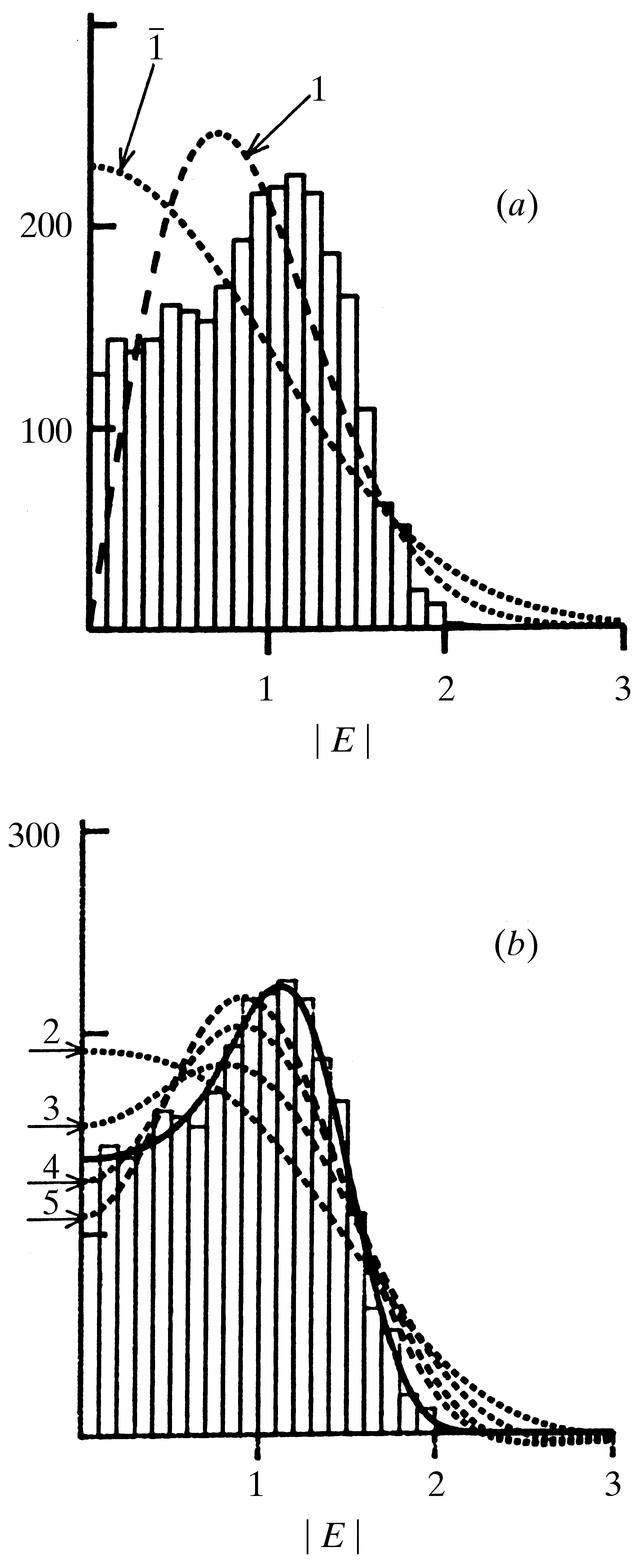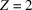International
Tables for
Crystallography
Volume B
Reciprocal space
Edited by U. Shmueli

International Tables for Crystallography (2006). Vol. B. ch. 2.1, p. 199   | 1 | 2 |

## Figure 2.1.7.1

U. Shmuelia* and A. J. C. Wilsonb

aSchool of Chemistry, Tel Aviv University, Tel Aviv 69 978, Israel, and bSt John's College, Cambridge, England
Correspondence e-mail:  ushmueli@post.tau.ac.ilFigure 2.1.7.1 Atomic heterogeneity and intensity statistics. The histogram appearing in (a) and (b) was constructed fromvalues which were recalculated from atomic parameters published for the centrosymmetric structure of C6H18Cl2N4O4Pt (Faggiani et al., 1980). The space group of the crystal is,, i.e. all the atoms are located in general positions. (a) A comparison of the recalculated distribution ofwith the ideal centric [equation (2.1.5.11)] and acentric [equation (2.1.5.8)] p.d.f.'s, denoted byand 1, respectively. (b) The same recalculated histogram along with the centric correction-factor p.d.f. [equation (2.1.7.5)], truncated after two, three, four and five terms (dashed lines), and with that accurately computed for the correct space-group Fourier p.d.f. [equations (2.1.8.5)and (2.1.8.22)] (solid line).